# Support Vector Machine

SVM ( Support Vector Machines ) is a supervised machine learning algorithm which can be used for both classification and regression challenges. But, It is widely used in classification problems.

In SVM, we plot each data item as a point in n-dimensional space (where n = no of features in a dataset) with the value of each feature being the value of a particular coordinates. Such that, value of feature is equal to the value of coordinate then we perform classification by finding the most appropriate hyperplane that differentiates two classes very well.

# Goal in SVM

Our goal is to determine some establishing decision boundary which is capable of separating the data into homogeneous groups using the features provided.

In Support Vector Machine, Support Vectors are the data points that are closer to hyperplane and influence the position and orientation of hyperplane.

There can be two forms of data like data which is linearly separable and data which is not linearly separable.

In case of linearly separable data, SVM forms a hyperplane that segregate the data . Hyperplane is a decision boundary that help to classify data points. It is a subspace which consists of one less dimension than your feature space. for eg- In 2 dimensions or features, hyperplane is a straight line(2–1). and In 3 dimensions or features , hyperplane is a two-dimensional subspace(3–1). So, We can say that dimensions of a hyperplane depends upon the no of features in dataset. It is a generalization of plane.

Hyperplane is only build when the data points in your dataset are linear separable. Now, Let us understand How to derive an hyperplane and to see which side of the plane, we’re in exactly?

We will use the values of a features and consider it as a coordinate to construct a hyperplane. Let’s create a ‘B’ Hyperplane in 2 dimensional space with two different classes . Red with coordinates(5,5) and Green with coordinates(-5,0) as given below.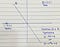Image1.o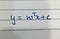Image2.o

In Image2.o, ‘W’ is a dot product of the parameters of the line , ‘X’ is the vector representation of coordinates and ‘C’ is the y intercept.

We ‘re transposing the ‘W’ vector to do the matrix multiplication with ‘X’. After doing the matrix multiplication. We will get the values either positive or negative.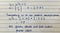Image3.0

In the above Image3.o, We classified all the green points as a positive class. So, We can say that if you take the dot product of weight vector and a vector itself with respect to data point than values comes out to be either positive or negative.

If it is with a positive sign then you’re on one side of the line and If the value is negative then you’re on the other side of the line. Finally, We get the projection of vector (-5,0) on the line. Same way, We can do it with other point(5,5) and project this point on the line.

We get the positive or negative values based on the dot product of transpose weight(W) and vector itself (X). These weight vectors corresponds to the hyperplane i.e. finding plane that best separates the data point. Now, We will be much better in performing classification problems.

In the above scenario, the line was passing directly from the origin. There was no shift in the line. But, what happens when we shift our line with some amount?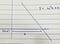Image4.o

In the above image4.o, Line is shifted by amount b. Label(y) will be predicted in a similar manner i.e. If the dot product is positive then we may say that this label is positive (+1). and if it is negative we say it as negative(-1) label.

Now, understand the above scenario in a detailed manner. This helps us to do analyse the internal working of finding the best hyperplane by applying Maximum and Soft Margin techniques.

# Maximum Margin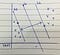Image5.o

In the above Image5.o , We want L1 line right in the center in order to segregate the data points in a efficient way. We will draw L2 and L3 line on both the sides of the L1 line. It’s like L1 line is stretching its arms on both the sides and having an idea about how far it can go. It is trying to adjust itself by setting L2 and L3 line towards both the side of its class. As soon as L2 line encountered with its first positive class. It will stop there ,same goes with L3 line when it will reach its first negative class i.e. green. So equation of L2 and L3 line is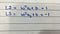Image6.o

In Imge6.o, It can be anything in place of 1 and -1, as these constraints won’t matter. What really matters is two different classes established i.e. positive and negative. We are trying to find out the distance between L2 and L3 line. So, to calculate the maximum distance between two lines . We will subtract the equations of both the lines.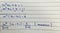image7.o

Above equation will get normalized by the norm of W i.e. weight vector because our main aim is to get rid of the transpose weight.

Now, We want distance between lines L2 and L3 to be maximum i.e. 2/||w||. But, We can’t maximize it as much as we want. We need to stop at some point and the point where we need to stop is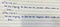Image8.o

Our main aim to do all this because we are trying to make space for L1 line to perform best segregation. But , We are limited by the above two conditions. If the above condition doesn’t hold true. then we will end up making wrong predictions.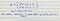Image9.o

It will optimize the value in such a way to make the correct predictions. In this case, We are trying to overfit with our training data. So to avoid overfitting in such scenarios we use Soft margin.

# How to avoid overfitting in SVM?

In SVM to avoid overfitting, We choose a soft margin, instead of a hard one i.e. We let some data points to enter our margin deliberately(but we still penalize it) so that our classifier don’t overfit on our training samples.

# Soft Margin

Using the Maximum Margin like in the above case, We somehow overfit with our current training data. Sometimes there would be situations that we want to relax our model a little. Not only prioritize to make correct predictions.

So, taking the above maximum margin equation value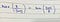Image10.o

Now, the point comes is Why we ‘re minimizing?

Like in Gradient descent, We use Derivatives to minimize the error function same here also , We can find derivatives to minimize i.e. derivatives on the curve or equation. So Max(2/||w||) = Min(2/||w||)

There is a need to relax our model so that our model not only performs well on training but also perform well in real scenarios, otherwise We’ll end up overfitting. We will be using the equation as shown in image11.o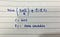Image11.o

Here , We are using some of the Regularization parameters like C, Ei (Slack Variable).

# Cost

C stands for cost i.e. how many errors you should allow in your model. C is 1 by default and its reasonable default choice. If you have a lot of noisy observations, you should decrease the value of C. It corresponds to the regularization of the hyperplane or you can also say, It smoothens the curve of the hyperplane.

In some scenarios, when cost is getting low, model will compromise on error and when cost is getting high, model will be judicious in making errors

# Ei (Slack Variable)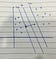Image12.o

Like in Image12.o, Slack Variable is the difference between misclassified points and the boundary of the line. So we are doing sum of all the errors that you allowed your model to make.

# Gamma

Gamma defines how far the influence of a single training observation reaches. If gamma has low values , It means far data points from the hyperplane has a high weightage when compare to data points nearby hyperplane. In this case, We may have a decision boundary linear, smoother and less draggy

If gamma has high values then data points nearby hyperplane has a higher weightage when compare to data points having far reach. In this case, decision boundary is more draggy.

So, higher value of gamma, will try to exact fit as per the training dataset which can cause generalization error and overfitting problem.

We should always look at the cross validation score to have effective combination of these parameters and avoid overfitting.

Now, What happens when in case of overlapping data points, no matter where we put our classifier . We will end up with a lot of misclassification. For eg, We have two categories but not obvious linear classifier that segregate the data in a efficient way. Since, we can say that data points are not linearly separable, So to make our model work , We use some of the kernels to move the data from lower dimensions to higher dimensions. Later, finding classifier that separates the higher dimension data into two groups. There are some of the kernel functions like polynomial and radial kernel that helps to find the classifier in higher dimensions.

These kernel functions calculate the relationship between every pair of points as they are in the higher dimensions. Calculating high dimensional relationship without transforming data to higher dimensions is called kernel trick. kernel trick reduces the amount of computation required for SVM that transform the data from low to high dimensions.

# Polynomial Kernel

Polynomial kernel, has a parameter ‘d’ which stands for the degree of polynomial. When d = 1, polynomial kernel computes the relationship between each pair of observation in 1 dimension. These relationships are used to find the classifier, When d = 2 , we get a 2d dimension based on x axis squared on the y axis. As the value of d increases, We compute the relationship between each pair of observation with increasing dimensions.

So, Polynomial kernel systematically increases dimensions by setting ‘d’ i.e. degree of polynomial relationship between each pair of observation to find classifier. We can have a good value of ‘d’ with CV.

Equation of a polynomial kernel is shown in Image13.o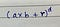Image13.o

a and b refers to two different observations in a dataset, r is the coefficient of the Polynomial and d is the degree of polynomial.

As we compute this equation, We get the dot product which gives us the high dimensional coordinates for the data. In order to calculate the high dimensional relationships, We calculate the dot product between each pair of data points.

# Radial Kernel

Radial kernel finds classifier in infinite dimensions, it’s not possible to visualize what it does. But with every new observation, RBF behaves like a weighted nearest weight(closest observation have a lot of influence on how we classify). So, RBF Kernel determines how much influence each observation has in the training dataset on classifying new observations. Equation of a Radial kernel is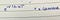Image14.o

a and b are the data points of two different classes. Amount of influence one observation has on another is a function of squared distance. Gamma scales the squared distance and thus it scales the influence.

So, by scaling the distance , Gamma scales the amount of influence two points have on each other. Just like in polynomial kernel, when we plug values in a radial kernel we will get the dot product. This dot product are the new coordinates in the in infinite no of dimensions. Equation of a radial kernel is similar to the Taylor series then only we get the infinite no of dimensions.

SVM Advantages

SVM’s are very good when we have no idea on the data.

Works well with even unstructured and semi structured data like text, images.

It scales relatively well to high dimensions.

SVM Models are generalized. Risk of overfitting is less in SVM

SVM Disadvantages

Choosing a good kernel function is not easy.

Long training time for large datasets.

It is not easy to tune the hyperparameters like cost and gamma . It is hard to visualize their impact.